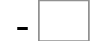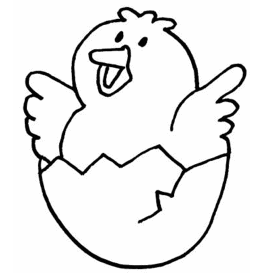1= 0   6= 17= 3   7= 4   10= 3   9= 7 8= 6   10= 3   9= 3   4= 0